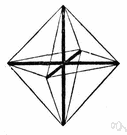# affine transformation

(redirected from Affine mapping)
Also found in: Thesaurus, Encyclopedia.
ThesaurusAntonymsRelated WordsSynonymsLegend:
 Noun 1affine transformation - (mathematics) a transformation that is a combination of single transformations such as translation or rotation or reflection on an axismath, mathematics, maths - a science (or group of related sciences) dealing with the logic of quantity and shape and arrangementtransformation - (mathematics) a function that changes the position or direction of the axes of a coordinate system
Based on WordNet 3.0, Farlex clipart collection. © 2003-2012 Princeton University, Farlex Inc.
References in periodicals archive ?
We say that C has the fixed point property for continuous affine mappings if every continuous affine mapping T : C [right arrow] C has a fixed point.
Let [PSI] be an affine mapping in [R.sup.3], obtained by composing a translation, an orthogonal transformation, and a scaling, as shown in Example 3, so that [PSI]([p.sub.0]) = (0, 0,0) and [PSI]([p.sub.0]) = (1,0,0).
(a) Suppose [[a.sub.1]; [a.sub.2]]; [[b.sub.1]; [b.sub.2]] [member of] (CC ([R.sup.1]) ; h) : Then h([[a.sub.1]; [a.sub.2]]; [[b.sub.1]; [b.sub.2]]) = max{|[b.sub.1] [a.sub.1]| ; |[b.sub.2] [a.sub.2]|}; and the mapping T : (CC [R.sup.1]) ; h) [right arrow] ([R.sup.1]; |*|) defined by T([[a.sub.1];[a.sub.2]]) = [a.sub.2] is a continuous (in fact, nonexpansive), affine mapping.
[B.sub.2] is not obtained from [B.sub.1] by an affine mapping. There is no exact similarity between these two curves.
In , another S-box is developed based on affine mapping having 253 nonzero terms in its polynomial and reuses the existing implementation of AES.
DIR was implemented with MI lung mask affine mapping combined with CC lung diffeomorphic (greedy SyN) mapping using the advanced normalization tools (ANTs) software package (open-source software; http://picsl.upenn.edu/software/ants).
For each [R.sub.i], a contractive affine mapping [T.sub.i] is searched in all domain blocks to find the closest [D.sub.j].
With above notations, let g : [R.sup.2] [right arrow] [R.sup.2] be an affine mapping,
From other methods that can be used in this context we would mention affine mapping on a triangulation and piecewise conformal mapping on convex polygons.

Site: Follow: Share:
Open / Close